# To determine:To Find:the measure of side VC in the figure shown such that VA' = 15, A'A=20 and VC'=18 Given: Figure is shown below. 12210202971.jpgQuestion
SimilarityTo determine:To Find:the measure of side VC in the figure shown such that VA' = 15, A'A=20 and VC'=18
Given:
Figure is shown below.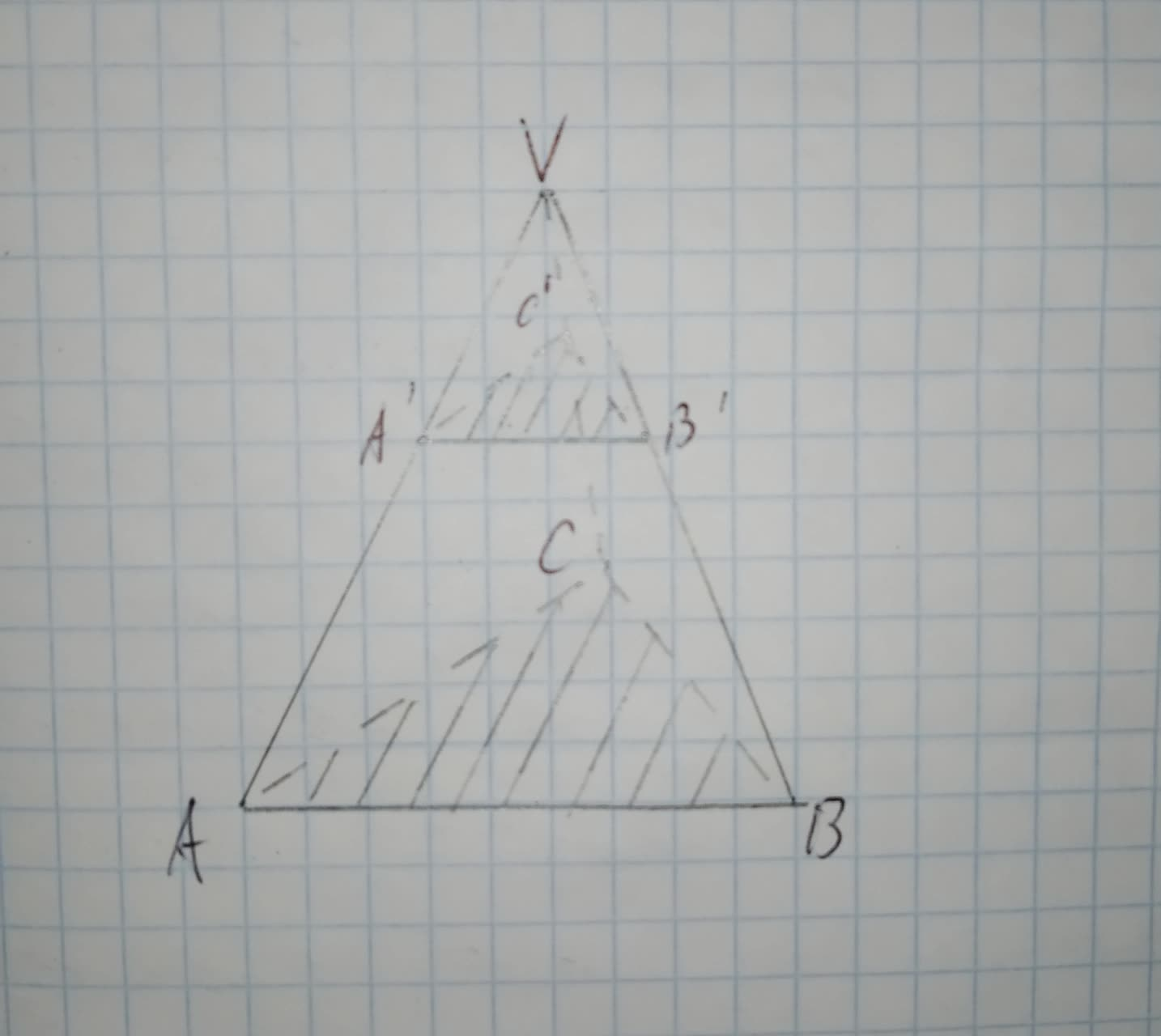2021-01-16
Calculation:
Since, plane of $$\displaystyle\triangle{A}'{B}'{C}'$$ is similar to the plane of $$\displaystyle\triangle{A}{B}{C}$$
Since, ABCD is a parallelogram.
$$\displaystyle\angle{B}=\angle{V}\therefore$$(Common)
$$\displaystyle\angle{V}{A}{B}=\angle{V}{A}'{B}'\therefore$$(Corresponding angle in two similar plane)
Therefore by AA similarity, $$\displaystyle\triangle{V}{A}{B}\sim\triangle{V}{A}'{B}'$$
Ratio of corresponding sides in two similar triangles is equal.
$$\displaystyle\frac{{{V}{A}}}{{{V}{A}'}}=\frac{{{V}{C}}}{{{V}{C}'}}$$
$$\displaystyle\frac{{{V}{A}'+\forall'}}{{{V}{A}'}}=\frac{{{V}{C}}}{{{V}{C}'}}$$
$$\displaystyle\frac{{{15}+{20}}}{{{15}}}=\frac{{{V}{C}}}{{{18}}}$$
$$\displaystyle{V}{C}=\frac{{{18}\times{35}}}{{{15}}}$$
VC=42

### Relevant QuestionsTo determine:To Find:the measure of side BB' in the figure shown such that VA' = 15, A'A=20 and A'B'=24
Given:
Figure is shown below.To determine:To Find:the measure of side BB' in the figure shown such that VA' = 15, A'A=20 and VB=49
Given:
Figure is shown below.To Complete:the statement WZ=? and RS=? in the figure shown.
Given:
Figure is shown below.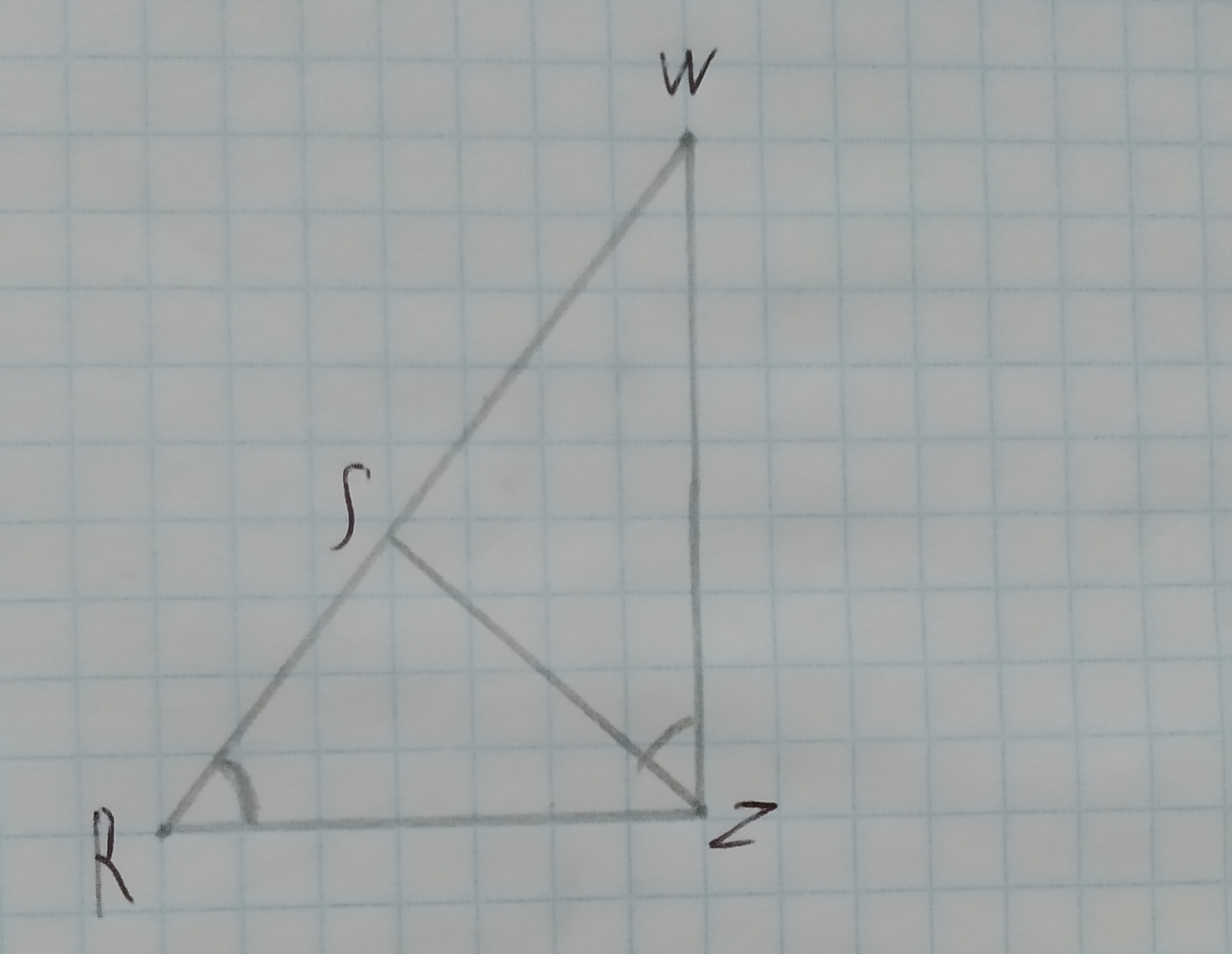RW=15, ZR=10 and ZS=8To Complete: the statement $$\displaystyle\frac{{{R}{W}}}{?}=\frac{{{Z}{R}}}{?}=\frac{{{W}{Z}}}{?}$$ in the figure shown,
Given:
Figure is shown below.To Complete: the statement $$\displaystyle\triangle{R}{W}{Z}=$$? in the figure shown.
Given:
Figure is shown below.To write: the name of the postulate, that justifies the given statement.
Given information:In $$\displaystyle\triangle{A}{V}{B}{\quad\text{and}\quad}\triangle{N}{V}{K},\angle{A}=\angle{N}={60}^{{\circ}}$$.
The given figure is as follows: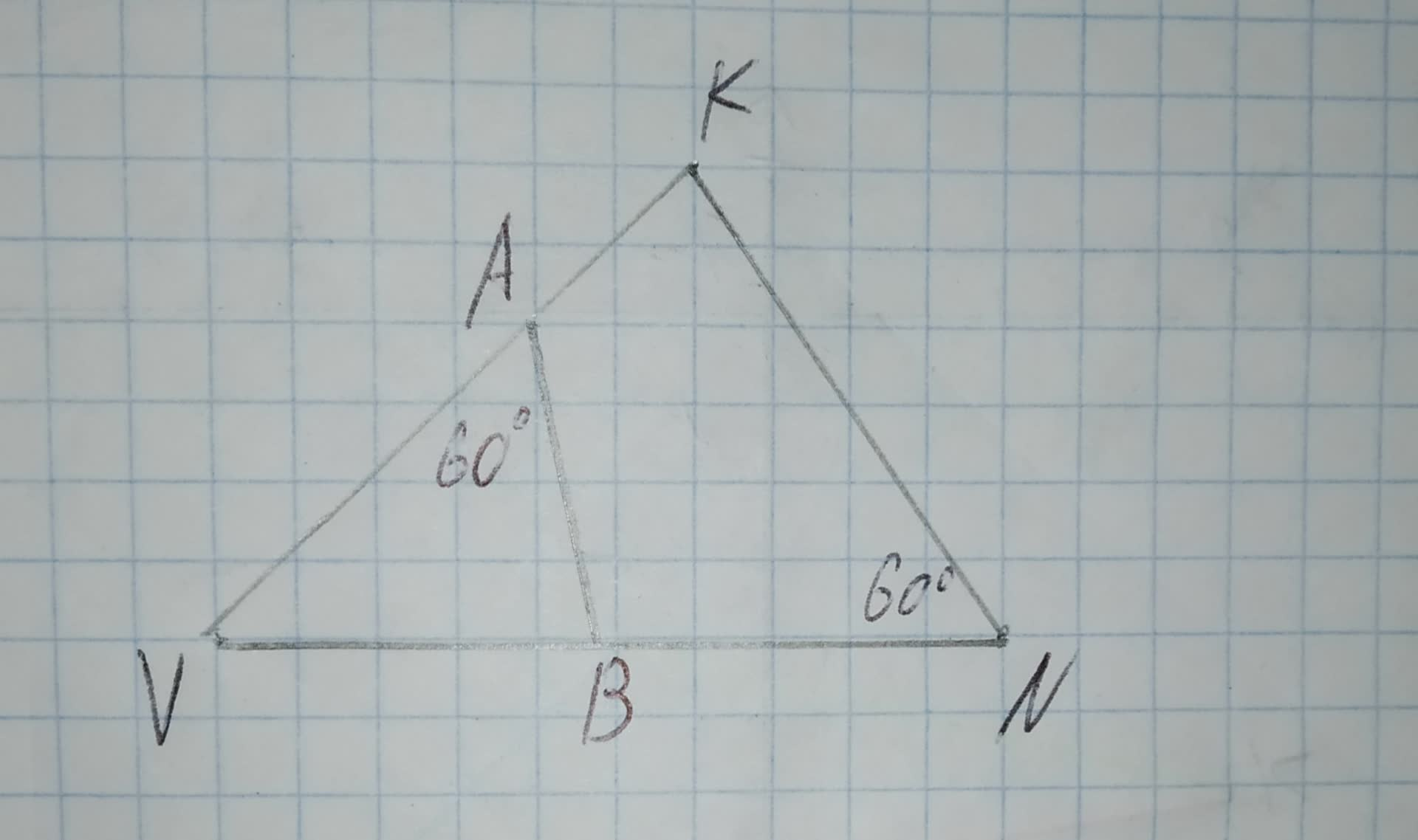To determine:Whether each pair of figures is similar and also write similarity statement and scale factor.
Given information:
Given the figure.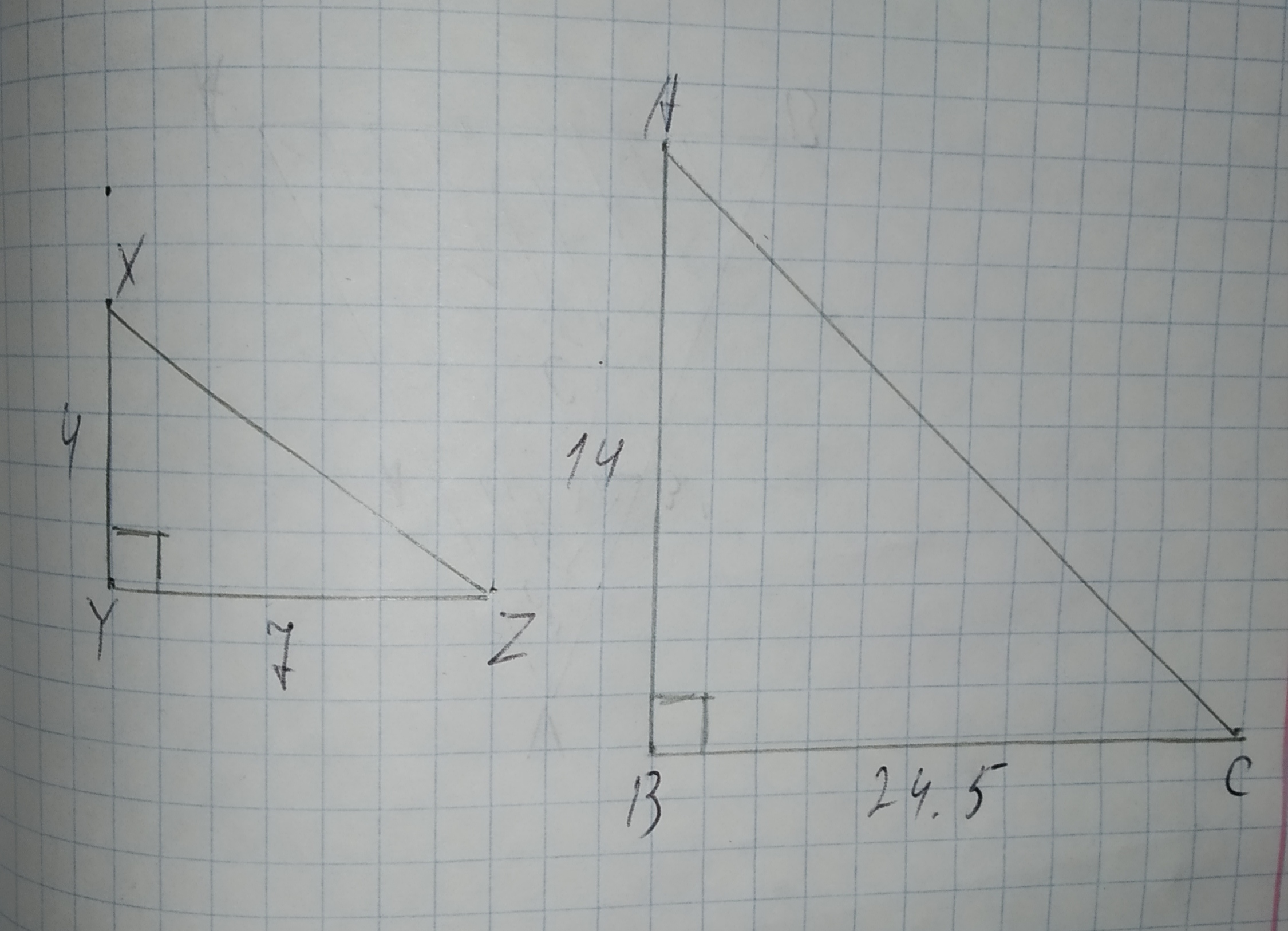To determine: The ratio of the sides of the triangles ABC and GHI.
Given:
Triangle ABC that is 75% of its corresponding side in triangle DEF.
Triangle GHI that is 32% of its corresponding side in triangle DEF.To determine: Whether the triangle ABC and GHI are similar to each other.
Given:
Triangle ABC that is 75% of its corresponding side in triangle DEF.
Triangle GHI that is 32% of its corresponding side in triangle DEF.Triangle MPT with $$\displaystyle\overline{{{N}{R}}}{\mid}{\mid}\overline{{{M}{T}}}$$ is shown below. The dimensions are in centimeters.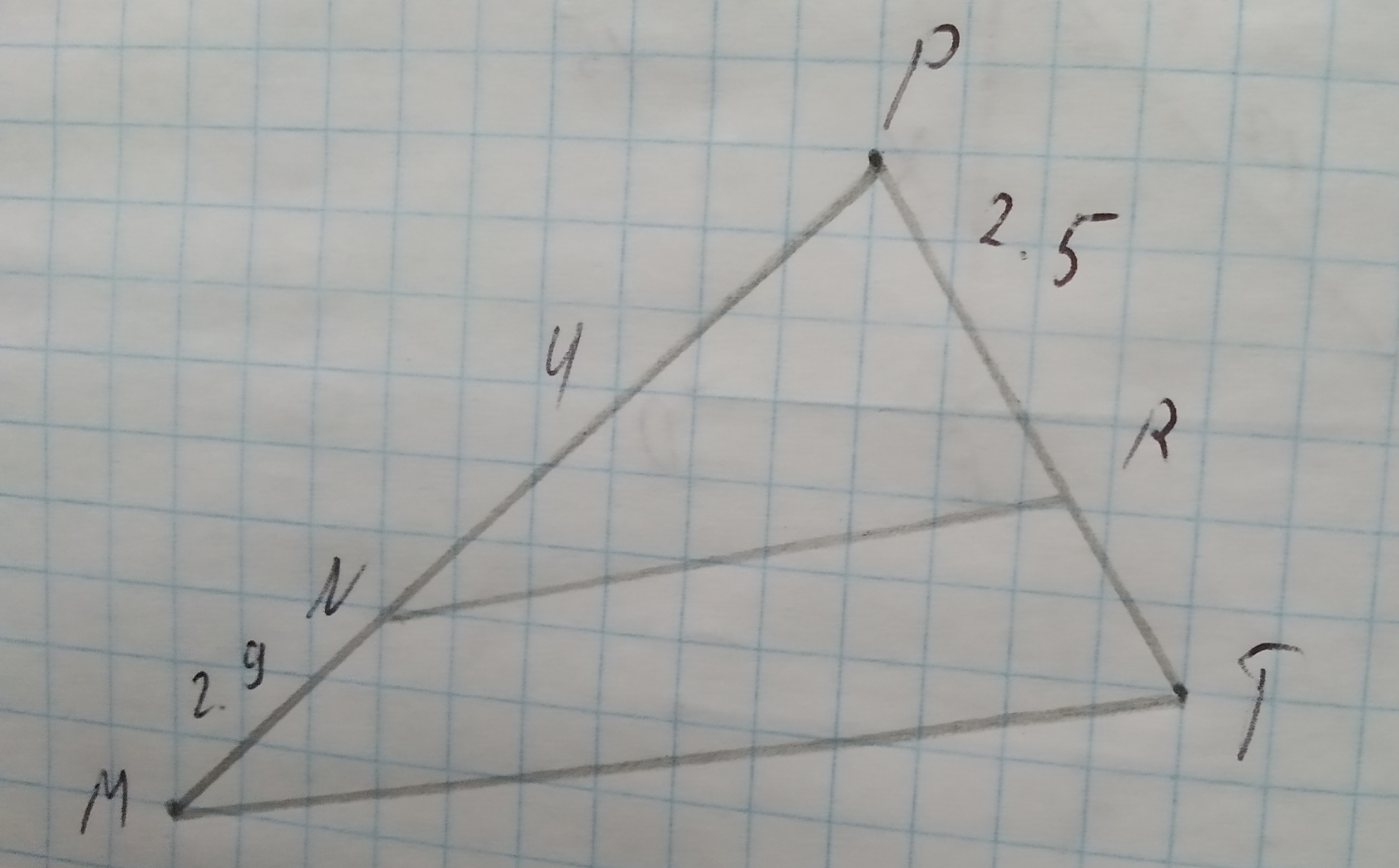Which measurement is closest to the length of $$\displaystyle\overline{{{R}{T}}}$$ in centimeters?